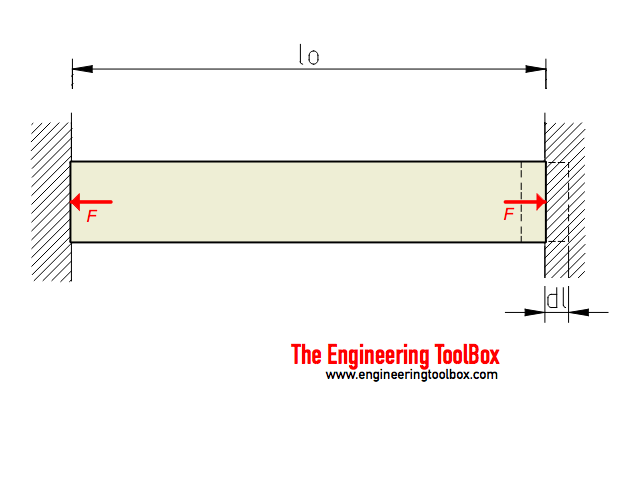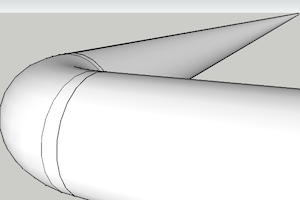Engineering ToolBox - Resources, Tools and Basic Information for Engineering and Design of Technical Applications!

# Restricted Thermal Expansion - Force and Stress

## Stress and force when thermal expansion a pipe, beam or similar is restricted.Linear expansion due to change in temperature can be expressed as

dl = α lo dt                              (1)

where

dl = elongation (m, in)

α = temperature expansion coefficient (m/mK, in/in oF)

lo = initial length (m, in)

dt = temperature difference (oC, oF)

The strain - or deformation - for an unrestricted expansion can be expressed as

ε = dl / lo                                  (2)

where

ε = strain - deformation

The Elastic modulus (Young's Modulus) can be expressed as

E = σ ε                                (3)

where

E = Young's Modulus (Pa (N/m2), psi)

σ = stress (Pa (N/m2), psi)

### Thermal Stress

When restricted expansion is "converted" to stress - then (1), (2) and (3) can be combined to

σdt = E ε

= E  dl / lo

= E α lo dt / lo

= E α dt                                  (4)

where

σdt = stress due to change in temperature (Pa (N/m2), psi)

### Axial Force

The axial force acted by the restricted bar due to change in temperature can be expressed as

F = σdt

E α dt A                                  (5)

where

F = axial force (N)

A = cross-sectional area of bar (m2, in2)

#### Example - Heated Steel Pipe - Thermal Stress and Force with Restricted Expansion

A DN150 Std. (6 in) steel pipe with length 50 m (1969 in) is heated from 20oC (68oF) to 90oC (194oF). The expansion coefficient for steel is 12 10-6 m/mK (6.7 10-6 in/inoF). The modulus of elasticity for steel is 200 GPa (109 N/m2) (29 106 psi (lb/in2)).make 3D models with The Engineering ToolBox Sketchup Extension

Expansion of unrestricted pipe:

dl = (12 10-6 m/mK) (50 m) ((90oC) - (20oC))

= 0.042 m

If the expansion of the pipe is restricted - the stress created due to the temperature change can be calculated as

σdt(200 109 N/m2) (12 10-6 m/mK) ((90oC) - (20oC))

= 168 106 N/m2 (Pa)

= 168 MPa

Note! - if there is pressure in the pipe - the axial and circumferential (hoop) stress may be added to restricted temperature expansion stress by using vector addition.

The outside diameter of the pipe is 168.275 mm (6.63 in) and the wall thickness is 7.112 mm (0.28 in). The cross-sectional area of the pipe wall can then be calculated to

A = π ((168.275 mm) / 2)2 - π ((168.275 mm) - 2 (7.112 mm)) / 2)2

= 3598 mm2

= 3.6 10-3 m2

The force acting at the ends of the pipe when it is restricted can be calculated as

F = (168 106 N/m2) (3.6 10-3 m2)

= 604800 N

= 604 kN

##### The calculation in Imperial units

Expansion of unrestricted pipe:

dl = (6.7 10-6 in/inoF) (1669 in) ((194oF) - (68oF))

= 1.4 in

Stress in restricted pipe:

σdt(29 106 lb/in2) (6.7 10-6 in/inoF) ((194oF) - (68oF))

= 24481 lb/in2 (psi)

Cross sectional area:

A = π ((6.63 in) / 2)2 - π ((6.63 in) - 2 (0.28 mm)) / 2)2

= 5.3 in2

Axial force acting at the ends:

F = (24481 lb/in2) (5.3 in2)

= 129749 lb

### Example - Thermal Tensions in Reinforced or Connected Materials

When two materials with different temperature expansion coefficients are connected - as typical with concrete and steel reinforcement, or in district heating pipes with PEH insulation etc. - temperature changes introduces tensions.

This can be illustrated with a PVC plastic bar of 10 m reinforced with a steel rod.

The free expansion of the PVC bar without the reinforcement - with a temperature change of 100 oC - can be calculated from (1) to

dlPVC = (50.4 10-6 m/mK) (10 m) (100 oC)

= 0.054 m

The free expansion of the steel rod with a temperature change of 100 oC - can be calculated from (1) to

dlsteel = (12 10-6 m/mK) (10 m) (100 oC)

= 0.012 m

If we assume that the steel rod is much stronger than the PVC bar (depends on the Young's modulus and the areas of the materials) - the tension in the PVC bar can be calculated from the difference in temperature expansion with (4) as

σPVC = (2.8 109 Pa) (0.054 m - 0.012 m) / (10 m)

= 11.8 106 Pa

= 11.8 MPa

The Tensile Yield Strength of PVC is approximately 55 MPa.

### Thermal Expansion Axial Force Calculator

This calculator can be used to calculate the axial force caused by an object with restricted temperature expansion. The calculator is generic and can be used for both metric and imperial units as long as the use of units are consistent.

Length of restricted object (m, inches)

Area of restricted object (m2, in2)

Temperature difference (oC, oF)

Young's modulus (GPa, 109 psi)

Expansion coefficient  (10-6 m/mK, 10-6 in/inoF)

## Related Topics

• ### Mechanics

Forces, acceleration, displacement, vectors, motion, momentum, energy of objects and more.
• ### Statics

Loads - forces and torque, beams and columns.
• ### Temperature Expansion

Thermal expansion of pipes and tubes - stainless steel, carbon steel, copper, plastics and more.

## Related Documents

• ### Fixed Pipes - Stress vs. Change in Temperature

Temperature changes introduces stress fixed pipes.
• ### Fixed Temperature Points

Temperature points that can be used as a reference for calibration
• ### Linear Thermal Expansion

Online linear temperature expansion calculator.
• ### Metals - Temperature Expansion Coefficients

Thermal expansion coefficients metals.
• ### Metals and Alloys - Young's Modulus of Elasticity

Elastic properties and Young's modulus for metals and alloys like cast iron, carbon steel and more.
• ### Pipes and Tubes - Temperature Expansion

Pipes expands when heated and contracts when cooled and the expansion can be expressed with the expansion equation.
• ### Piping Elbows - Thrust Block Forces

Thrust block forces on pipe bends anchors due to liquid velocities and internal pressures - online resulting force calculator.
• ### Piping Materials - Temperature Expansion Coefficients

Temperature expansion coefficients for materials used in pipes and tubes like aluminum, carbon steel, cast iron, PVC, HDPE and more.
• ### Piping Materials - Temperature Heat Expansion and Cooling Contraction

Expansion and contraction when cast iron, carbon and carbon molybdenum steel, wrought iron, copper, brass and aluminum pipes are heated or cooled.
• ### Pressfit Pipes - Expansion Loops

Temperature expansion loops with pressfit piping.
• ### Shrink-Fits Assembly

Assembly temperatures for shrink-fits.
• ### Solids - Volume Temperature Expansion Coefficients

Cubical expansion coefficients for solids.
• ### Steam Pipes - Thermal Expansion

Thermal expansion of steam pipes heated from room temperature to operation temperature (mm pr. 100 m pipe).
• ### Steel Pipes - Calculate Thermal Expansion Loops

Calculating and sizing steel pipe thermal expansion loops.
• ### Steel Pipes - Temperature Expansion

Calculate temperature expansion with carbon steel pipes.
• ### Stress

Stress is force applied on cross-sectional area.
• ### Stress in Thick-Walled Cylinders or Tubes

Radial and tangential stress in thick-walled cylinders or tubes with closed ends - with internal and external pressure.
• ### Stress, Strain and Young's Modulus

Stress is force per unit area - strain is the deformation of a solid due to stress.
• ### Thermal Expansion - Linear Expansion Coefficients

Linear temperature expansion coefficients for common materials like aluminum, copper, glass, iron and many more.
• ### Thermal Expansion of Steam Pipes - inches

Expansion of steam pipes heated from room temperature to operation temperature.
• ### Thermoplastics - Physical Properties

Physical properties of thermoplastics like ABS, PVC, CPVC, PE, PEX, PB and PVDF.
• ### Young's Modulus, Tensile Strength and Yield Strength Values for some Materials

Young's Modulus (or Tensile Modulus alt. Modulus of Elasticity) and Ultimate Tensile Strength and Yield Strength for materials like steel, glass, wood and many more.

## Engineering ToolBox - SketchUp Extension - Online 3D modeling!

Add standard and customized parametric components - like flange beams, lumbers, piping, stairs and more - to your Sketchup model with the Engineering ToolBox - SketchUp Extension - enabled for use with older versions of the amazing SketchUp Make and the newer "up to date" SketchUp Pro . Add the Engineering ToolBox extension to your SketchUp Make/Pro from the Extension Warehouse !

We don't collect information from our users. More about

## Citation

• The Engineering ToolBox (2011). Restricted Thermal Expansion - Force and Stress. [online] Available at: https://www.engineeringtoolbox.com/stress-restricting-thermal-expansion-d_1756.html [Accessed Day Month Year].

Modify the access date according your visit.

9.19.12